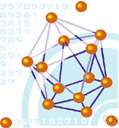molecular weight

(redirected from Weight-average molecular weight)
Also found in: Thesaurus, Medical, Acronyms, Encyclopedia.

molecular weight

n.
The sum of the atomic weights of all the atoms in a molecule. Also called relative molecular mass.

molecular weight

n
(Chemistry) the former name for relative molecular mass

molec′ular weight′

n.
the average weight of a molecule of an element or compound measured in units based on 1/12 the weight of the carbon-12 atom; the sum of the atomic weights of all the atoms in a molecule. Abbr.: mol. wt.
[1875–80]

molecular weight

The sum of the atomic weights of the atoms contained in a molecule. For example, since the atomic weight of hydrogen is 1 and the atomic weight of oxygen is 16, the molecular weight of water (H2O) is 1 + 1 + 16, or 18.
ThesaurusAntonymsRelated WordsSynonymsLegend:
 Noun 1molecular weight - (chemistry) the sum of the relative atomic masses of the constituent atoms of a moleculerelative molecular massmass - the property of a body that causes it to have weight in a gravitational fieldchemical science, chemistry - the science of matter; the branch of the natural sciences dealing with the composition of substances and their properties and reactions
Translations
References in periodicals archive ?
The weight-average molecular weight of the fluid in the (i + 1)th iterative step is calculated via Eq.
3, it can be seen that the weight-average molecular weight of the polymers increases quickly to a constant in the start-up process of the polymerization, and the plant data are lower than the simulated data.
The number-average molar mass of the polymer was 3.7 x [10.sup.5], and the weight-average molecular weight was 5.5 x the terpolymer began to decompose at 250[degrees]C.
where [[eta].sub.o] is the zero shear viscosity, [M.sub.w] is the weight-average molecular weight, and k is a constant that is specific to the polymer being evaluated.
The primary reactive functionality present in these oligomeric chain extenders is the epoxy groups, which react preferentially with carboxylic acid end-groups of PLA to generate esters of increased weight-average molecular weight and viscosity.
Weight-average molecular weight data are hardly ever reported (with the usual argument that the polydispersity index, PDI, is usually low and close to 1.1-1.2).
The membrane includes a polyolefin with a weight-average molecular weight of 5_105 or more or polyolefin fibrils with a weight-average molecular weight of 5_105 or more.
The most common reported value is Mw (weight-average molecular weight)
On an empirical basis, once the chains were of sufficient length, the residual orientation measured for each weight fraction was found to increase logarithmically with the weight-average molecular weight of the deuterated fraction.
developed a helical chiral polymer such as polylactic acid with a weight-average molecular weight of 50,000-1,000,000, optical activity, and 20-80% crystallinity, showing enhanced piezoelectricity.

Site: Follow: Share:
Open / Close